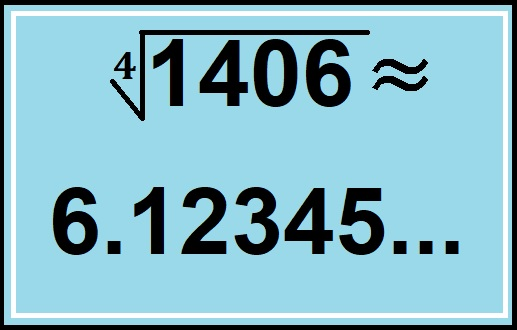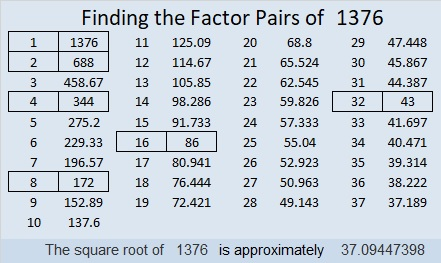# 1406 Has a Very Cool 4th Root

To find the 4th root of 1406, all you need to do is take its square root twice. The square root of 1406 is 37.4966665185. . .

Take the square root of that and you get a decimal starting with 6.12345…Here’s a little more about the number 1406:

• 1406 is a composite number.
• Prime factorization: 1406 = 2 × 19 × 37.
• 1406 has no exponents greater than 1 in its prime factorization, so √1406 cannot be simplified.
• The exponents in the prime factorization are 1, 1, and 1. Adding one to each exponent and multiplying we get (1 + 1)(1 + 1)(1 + 1) = 2 × 2 × 2 = 8. Therefore 1406 has exactly 8 factors.
• The factors of 1406 are outlined with their factor pair partners in the graphic below.1406 is the sum of the first 37 EVEN numbers because 37 × 38=1406.

1406 is the hypotenuse of a Pythagorean triple:
456-1330-1406 which is (12-35-37) times 38

# 1376 Let’s Get Ready for the Playful Math Carnival!

Too many people think that mathematics is a house of horrors, but there are plenty of bloggers out there, who know that done right, math is actually ALL fun and games. It is like a carnival! Every month, you can play at the Playful Math Education Blog Carnival, and it really is play! What does a playful math carnival look like? Go on over to see how Math Mama Writes… and puts on a fabulous March carnival!

I will be hosting this monthly carnival the last week of April! Why do I get to host it? I sent a message on twitter to Denise Gaskins who coordinates the carnival, and I requested the privilege. If you would like to host it in the future, let her know. She is always looking for blogs to host, and she will be very happy to hear from you.

In the meantime, you can help me with my carnival. If you blog about mathematics in a playful way that could benefit children who are somewhere between preschool to high school age, I would love to include your post in my carnival. The carnival is a FREE way to promote your post, so if you would like more traffic to your blog, submit a post using the link from Denise Gaskins’ website by Friday, April 19. Then before the end of the month, you will be able to enjoy the carnival even more because of your participation!Now I’ll tell you a little bit about the post number, 1376:

• 1376 is a composite number.
• Prime factorization: 1376 = 2 × 2 × 2 × 2 × 2 × 43, which can be written 1376 = 2⁵ × 43
• 1376 has at least one exponent greater than 1 in its prime factorization so √1376 can be simplified. Taking the factor pair from the factor pair table below with the largest square number factor, we get √1376 = (√16)(√86) = 4√86
• The exponents in the prime factorization are 5 and 1. Adding one to each exponent and multiplying we get (5 + 1)(1 + 1) = 6 × 2 = 12. Therefore 1376 has exactly 12 factors.
• The factors of 1376 are outlined with their factor pair partners in the graphic below.As mentioned in my previous post, 1376 is part of the three smallest consecutive numbers that have cube roots that can be simplified.